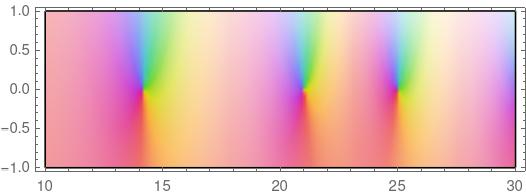# Bounding zeros of an analytic function

The previous post looked at the problem of finding the zeros of a cubic polynomial. Assuming we’re going to use a numerical method to calculate the zero, the hard part is knowing where to tell the numerical method to look. That post showed how to use a change of variables to guarantee that the polynomial has one, and only one, root in the unit interval.

Now suppose we’re looking at functions of a complex variable. On the real line we can say that if a function is negative here and positive there, it must be zero somewhere in between by the intermediate value theorem. But that’s not the case for a complex-valued function. Such a function can avoid going through zero because it can move in two dimensions, not just one.

For example, the function ez is never zero. Now eπi = -1 and e0 1, but there’s no point on a line from πi to 0 where the exponential function is zero.

So how can we tell whether an analytic function has a zero in some region of the complex plane? More generally, can we tell how many zeros it has in some region?

There is a theorem in complex analysis called the argument principle. It says that if an analytic function f is not zero along a closed curve C, then the number of zeros of f inside C equalsWe can evaluate this integral numerically, and it will tell us the number of zeros inside. The exact value must be an integer, so the integral doesn’t have to be computed with much accuracy. If we know the error is less than a half, then rounding the result to the nearest integer gives the exact answer.

## Riemann zeta example

Let’s use the argument principle to show that the Riemann zeta function ζ(z) has 3 zeros in the critical strip with imaginary part between 10 and 30. The critical strip is the part of the complex plane with real part between 0 and 1.

We can make our contour a rectangle with lower left corner at 10i and upper right corner at 1 + 30i.

We’ll use Mathematica and start by defining a function leg that numerically integrates along one leg of a rectangle.

    leg[z1_, z2_] := NIntegrate[Zeta'[z]/Zeta[z], {z, z1, z2}]

Now let’s use this function to integrate over the rectangle described above.

    (leg[0+10I, 1+10I] + leg[1+10I, 1+30I] +
leg[1+30I, 0+30I] + leg[0+30I, 0+10I]) / (2 Pi I)


This returns

    3. +3.77069*10^-12 I

and the nearest integer is 3, confirming that the zeta function has 3 zeros inside our box, assuming that our numerical integration error is less than 0.5.

Next, let’s show that zeta has a zero in the critical strip with imaginary part between 14 and 15.

The integration

    (leg[0+14I, 1+14I] + leg[1+14I, 1+15I] +
leg[1+15I, 0+15I] + leg[0+15I, 0+14I]) / (2 Pi I)


returns

    1. + 1.46221*10^-12 I

showing that there’s one zero inside this smaller box.

In fact the first three zeros in the critical strip are 1/2 + 14.1347i, 1/2 + 21.0220i, and 1/2 + 25.0109i. The next one is at 1/2 + 30.4249i, just outside the first box we used above.

Here’s a plot.I plotted ζ(1/2 + iz) to turn the plot sideways. Here’s the code that produced the plot.

    ComplexPlot[Zeta[I z + 1/2], {z, 10 - I, 30 + I},
ColorFunction -> "LocalMaxAbs", AspectRatio -> 1/3]


More on plots of a complex function and what the colors mean here.